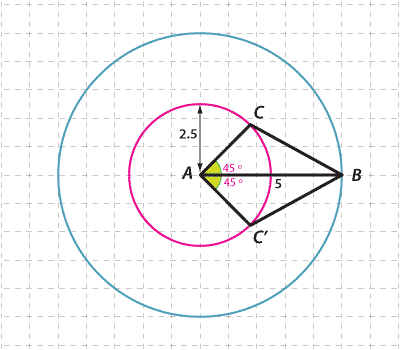### The SAS congruence test

• If two sides and an included angle of one triangle are respectively equal to two sides and the included angle of another triangle, then the triangles are congruent.
• If we are given the lengths of two sides of a triangle and the size of the included angle, then only one such triangle can be constructed (up to congruence).

The initials SAS stand for 'Side', 'Angle', 'Side'.

In the diagram below, $$AB$$ is 5 units in length:

• A pink circle is drawn with centre A and radius 2.5 units.
• AC is drawn so that $$\angle CAB$$ is 45 °.
• AC′ is drawn so that $$\angle C′AB$$ is 45 °.

The positions of C and C′ are fixed.Detailed description

#### Question 4

Construct a triangle with side lengths 6 cm and 4 cm with the angle between these sides being 50 ° by either using GeoGebra or other geometry packages, or using a compass and ruler.